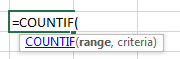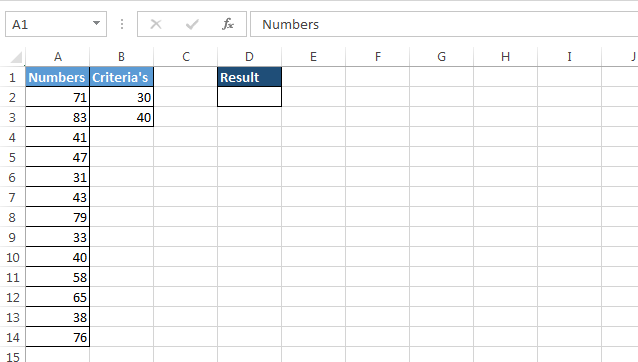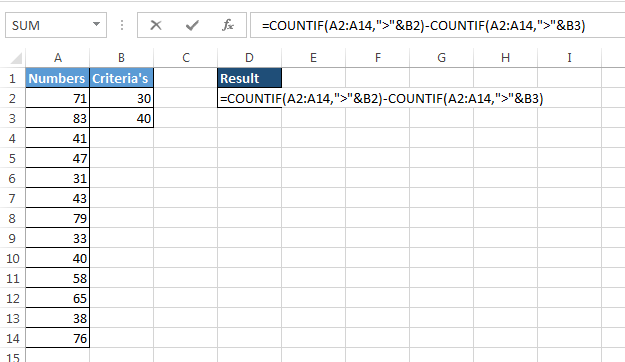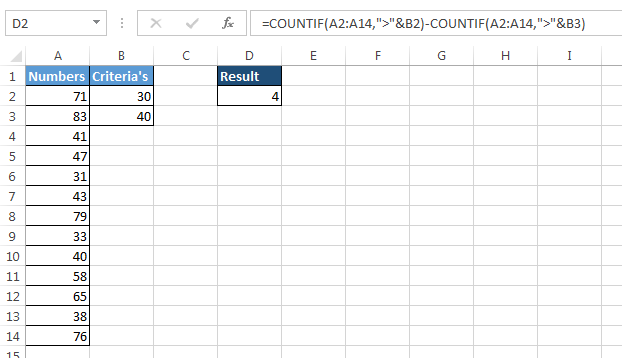# Count how many numbers are in series of numbers when a certain criteria is set in Microsoft Excel

In this article, we are going to learn how to count the numbers or a series of numbers when a certain criterion is set in Microsoft Excel. To solve this problem, we use COUNTIF function.

What is COUNTIF function?

On the basis of condition, to count the numbers within a range, we use COUNTIF function.How we will use COUNTIF function to count the number in series of numbers on the basis of criteria?

Let’s take an example to understand:-

We have numbers in column A & Criteria are set in cell B2, B3.• Enter the formula in cell D2

=COUNTIF(A2:A11,">"&B2)-COUNTIF(A2:A11,">"&B3)• Press Enter
• The function will return the numbers which will be falling under the criteria.Formula Explanation:-

• COUNTIF(A2:A11,">"&B2), we will get 13 as the output
• COUNTIF(A2:A11,">"&B3), we will get 9 as the output
• Hence, 4 (13-9) is our output.